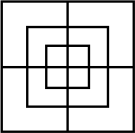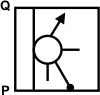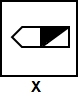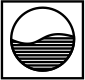# CREST Reasoning Olympiad Class 8 Sample Papers

REGISTER NOW

## Syllabus:

Section 1: Series Completion and Inserting the Missing Character, Analogy and Classification, Blood Relations, Coding-Decoding, Direction Sense Test, Logical Venn Diagram, Alpha Numeric Sequence Puzzle, Number, Ranking and Time Sequence Test, Logical Sequence of Words, Alphabet Test, Mathematical Operations, Analytical Reasoning, Mirror Images and Water Images, Embedded Figures, Figure Formation, Construction of Squares, Grouping of Identical Figures, Figure Matrix, Paper Folding and Paper Cutting, Cubes and Dice, Dot Situations.

Achievers Section: Higher Order Thinking Questions - Syllabus as per Section 1

 Q.1 Raj is the brother of Akhil. Ritika is the sister of Sohan. Akhil is the son of Ritika. Akhil is the son of Ritika. How is Raj related to Ritika?
 Q.2 If 'red' is called 'pink', 'pink' is called 'black', 'black' is called 'white' and 'white' is called 'blue', then colour of milk is _____________.
 Q.3 How many squares are there in the following figure?Q.4 If 'P' denotes 'multiplied by', 'T' denotes 'subtracted from', 'M' denotes 'added to' and 'B' denotes 'divided by' then, what should be the correct response of 12 P 6 M 15 T 16 B 4?
 Q.5 Which of the following answer figures is exactly the mirror image of the question figure when the mirror is held on the line PQ?Q.6 KLM is related to MLK in the same way HIJ is related to
 Q.7 In the following question, choose the correct mirror image of the given figure (X), if a mirror is placed vertically on the left side of the figure.Q.8 Select the pair which shows similar relationship that is shown by the following pair.ABC : CDE
 Q.9 A is South-West of B, C is the South-East of B, then C is in which direction of A?
 Q.10 From the given suitable options, find the water image of the object given in the question figure.Sample PDF of CREST Reasoning Olympiad for Class 8: